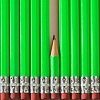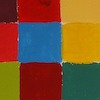# Angles, Polygons and Geometrical Proof (age 14-16)

This is part of our Secondary Curriculum collection of favourite rich tasks arranged by topic.##### Age 11 to 16Challenge Level

Draw some quadrilaterals on a 9-point circle and work out the angles. Is there a theorem?### Triangle Midpoints

##### Age 14 to 16Challenge Level

You are only given the three midpoints of the sides of a triangle. How can you construct the original triangle?### Making Sixty

##### Age 14 to 16Challenge Level

Why does this fold create an angle of sixty degrees?##### Age 14 to 16Challenge Level

Explore when it is possible to construct a circle which just touches all four sides of a quadrilateral.### Nicely Similar

##### Age 14 to 16Challenge Level

If the hypotenuse (base) length is 100cm and if an extra line splits the base into 36cm and 64cm parts, what were the side lengths for the original right-angled triangle?##### Age 14 to 16Challenge Level

Two ladders are propped up against facing walls. The end of the first ladder is 10 metres above the foot of the first wall. The end of the second ladder is 5 metres above the foot of the second wall. At what height do the ladders cross?### Sitting Pretty

##### Age 14 to 16Challenge Level

A circle of radius r touches two sides of a right angled triangle, sides x and y, and has its centre on the hypotenuse. Can you prove the formula linking x, y and r?### Kite in a Square

##### Age 14 to 18Challenge Level

Can you make sense of the three methods to work out what fraction of the total area is shaded?### Trapezium Four

##### Age 14 to 16Challenge Level

The diagonals of a trapezium divide it into four parts. Can you create a trapezium where three of those parts are equal in area?### Partly Circles

##### Age 14 to 16Challenge Level

What is the same and what is different about these circle questions? What connections can you make?### Napkin

##### Age 14 to 16Challenge Level

A napkin is folded so that a corner coincides with the midpoint of an opposite edge . Investigate the three triangles formed .### Angle Trisection

##### Age 14 to 16Challenge Level

It is impossible to trisect an angle using only ruler and compasses but it can be done using a carpenter's square.### Squirty

##### Age 14 to 16Challenge Level

Using a ruler, pencil and compasses only, it is possible to construct a square inside any triangle so that all four vertices touch the sides of the triangle.### Same Length

##### Age 11 to 16Challenge Level

Construct two equilateral triangles on a straight line. There are two lengths that look the same - can you prove it?### Angles, Polygons and Geometrical Proof Short Problems

##### Age 11 to 16

A collection of short problems on Angles, Polygons and Geometrical Proof.### Isosceles Seven

##### Age 14 to 16Challenge Level

Is it possible to find the angles in this rather special isosceles triangle?### The Square Under the Hypotenuse

##### Age 14 to 16Challenge Level

Can you work out the side length of a square that just touches the hypotenuse of a right angled triangle?